# 3.7 Inverse functions  (Page 8/9)

 Page 8 / 9

$f\left(x\right)=-3x+5$

one-to-one

$f\left(x\right)=|x-3|$

For the following exercises, use the vertical line test to determine if the relation whose graph is provided is a function.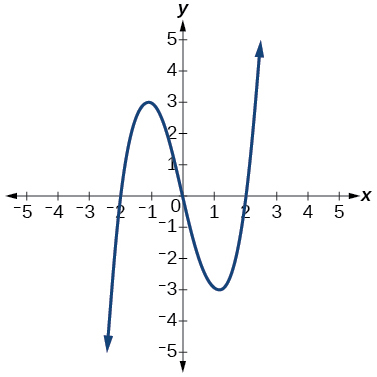function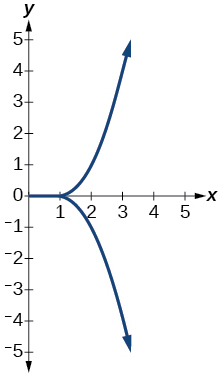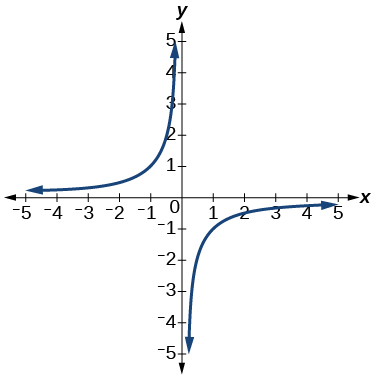function

For the following exercises, graph the functions.

$f\left(x\right)=|x+1|$

$f\left(x\right)={x}^{2}-2$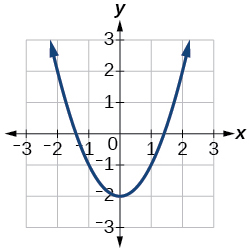For the following exercises, use [link] to approximate the values.

$f\left(2\right)$

$f\left(-2\right)$

$2$

If $\text{\hspace{0.17em}}f\left(x\right)=-2,\text{\hspace{0.17em}}$ then solve for $\text{\hspace{0.17em}}x.$

If $\text{\hspace{0.17em}}f\left(x\right)=1,\text{\hspace{0.17em}}$ then solve for $\text{\hspace{0.17em}}x.$

or

For the following exercises, use the function $\text{\hspace{0.17em}}h\left(t\right)=-16{t}^{2}+80t\text{\hspace{0.17em}}$ to find the values in simplest form.

$\frac{h\left(2\right)-h\left(1\right)}{2-1}$

$\frac{h\left(a\right)-h\left(1\right)}{a-1}$

$\frac{-64+80a-16{a}^{2}}{-1+a}=-16a+64$

## Domain and Range

For the following exercises, find the domain of each function, expressing answers using interval notation.

$f\left(x\right)=\frac{2}{3x+2}$

$f\left(x\right)=\frac{x-3}{{x}^{2}-4x-12}$

$\left(-\infty ,-2\right)\cup \left(-2,6\right)\cup \left(6,\infty \right)$

$f\left(x\right)=\frac{\sqrt{x-6}}{\sqrt{x-4}}$

Graph this piecewise function: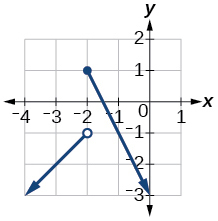## Rates of Change and Behavior of Graphs

For the following exercises, find the average rate of change of the functions from

$f\left(x\right)=4x-3$

$f\left(x\right)=10{x}^{2}+x$

$31$

$f\left(x\right)=-\frac{2}{{x}^{2}}$

For the following exercises, use the graphs to determine the intervals on which the functions are increasing, decreasing, or constant.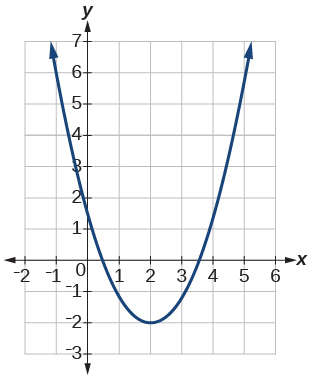increasing $\text{\hspace{0.17em}}\left(2,\infty \right);\text{\hspace{0.17em}}$ decreasing $\text{\hspace{0.17em}}\left(-\infty ,2\right)$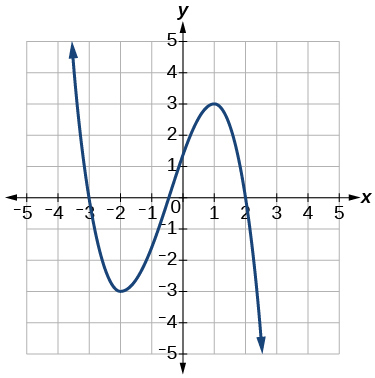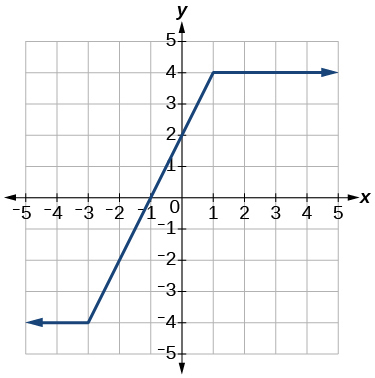increasing $\text{}\left(-3,1\right);\text{}$ constant $\text{\hspace{0.17em}}\left(-\infty ,-3\right)\cup \left(1,\infty \right)$

Find the local minimum of the function graphed in [link] .

Find the local extrema for the function graphed in [link] .

local minimum $\text{\hspace{0.17em}}\left(-2,-3\right);\text{\hspace{0.17em}}$ local maximum $\text{\hspace{0.17em}}\left(1,3\right)$

For the graph in [link] , the domain of the function is $\text{\hspace{0.17em}}\left[-3,3\right].$ The range is $\text{\hspace{0.17em}}\left[-10,10\right].\text{\hspace{0.17em}}$ Find the absolute minimum of the function on this interval.

Find the absolute maximum of the function graphed in [link] .

$\text{\hspace{0.17em}}\left(-1.8,10\right)\text{\hspace{0.17em}}$

## Composition of Functions

For the following exercises, find $\text{\hspace{0.17em}}\left(f\circ g\right)\left(x\right)\text{\hspace{0.17em}}$ and $\text{\hspace{0.17em}}\left(g\circ f\right)\left(x\right)\text{\hspace{0.17em}}$ for each pair of functions.

$f\left(x\right)=4-x,\text{\hspace{0.17em}}g\left(x\right)=-4x$

$f\left(x\right)=3x+2,\text{\hspace{0.17em}}g\left(x\right)=5-6x$

$\left(f\circ g\right)\left(x\right)=17-18x;\text{\hspace{0.17em}}\left(g\circ f\right)\left(x\right)=-7-18x$

$f\left(x\right)={x}^{2}+2x,\text{\hspace{0.17em}}g\left(x\right)=5x+1$

$\left(f\circ g\right)\left(x\right)=\sqrt{\frac{1}{x}+2};\text{\hspace{0.17em}}\left(g\circ f\right)\left(x\right)=\frac{1}{\sqrt{x+2}}$

For the following exercises, find $\text{\hspace{0.17em}}\left(f\circ g\right)\text{\hspace{0.17em}}$ and the domain for $\text{\hspace{0.17em}}\left(f\circ g\right)\left(x\right)\text{\hspace{0.17em}}$ for each pair of functions.

$\left(f\circ g\right)\left(x\right)=\frac{1}{\sqrt{x}},\text{\hspace{0.17em}}x>0$

For the following exercises, express each function $\text{\hspace{0.17em}}H\text{\hspace{0.17em}}$ as a composition of two functions $\text{\hspace{0.17em}}f\text{\hspace{0.17em}}$ and $\text{\hspace{0.17em}}g\text{\hspace{0.17em}}$ where $\text{\hspace{0.17em}}H\left(x\right)=\left(f\circ g\right)\left(x\right).$

$H\left(x\right)=\sqrt{\frac{2x-1}{3x+4}}$

sample: $\text{\hspace{0.17em}}g\left(x\right)=\frac{2x-1}{3x+4};\text{\hspace{0.17em}}f\left(x\right)=\sqrt{x}$

$H\left(x\right)=\frac{1}{{\left(3{x}^{2}-4\right)}^{-3}}$

## Transformation of Functions

For the following exercises, sketch a graph of the given function.

$f\left(x\right)={\left(x-3\right)}^{2}$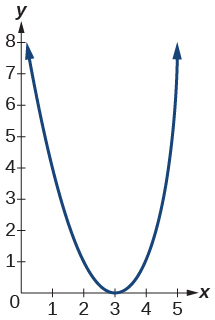$f\left(x\right)={\left(x+4\right)}^{3}$

$f\left(x\right)=\sqrt{x}+5$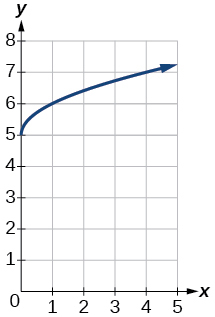$f\left(x\right)=-{x}^{3}$

$f\left(x\right)=\sqrt{-x}$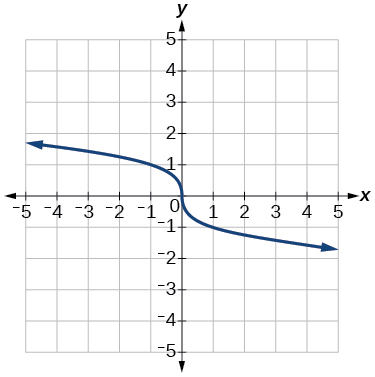$f\left(x\right)=5\sqrt{-x}-4$

$f\left(x\right)=4\left[|x-2|-6\right]$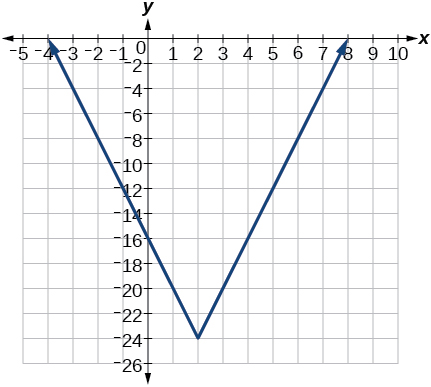$f\left(x\right)=-{\left(x+2\right)}^{2}-1$

For the following exercises, sketch the graph of the function $\text{\hspace{0.17em}}g\text{\hspace{0.17em}}$ if the graph of the function $\text{\hspace{0.17em}}f\text{\hspace{0.17em}}$ is shown in [link] .

$g\left(x\right)=f\left(x-1\right)$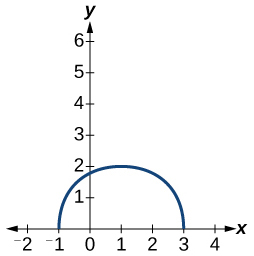$g\left(x\right)=3f\left(x\right)$

For the following exercises, write the equation for the standard function represented by each of the graphs below.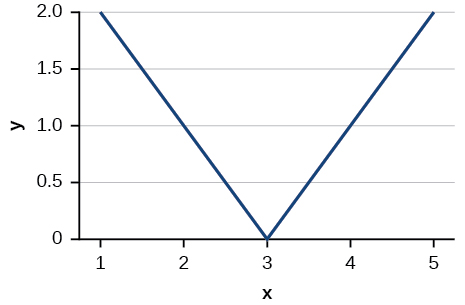$f\left(x\right)=|x-3|$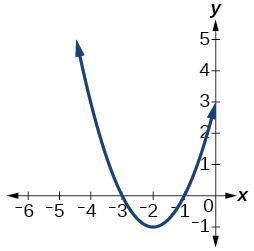For the following exercises, determine whether each function below is even, odd, or neither.

$f\left(x\right)=3{x}^{4}$

even

$g\left(x\right)=\sqrt{x}$

$h\left(x\right)=\frac{1}{x}+3x$

odd

For the following exercises, analyze the graph and determine whether the graphed function is even, odd, or neither.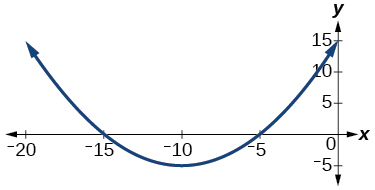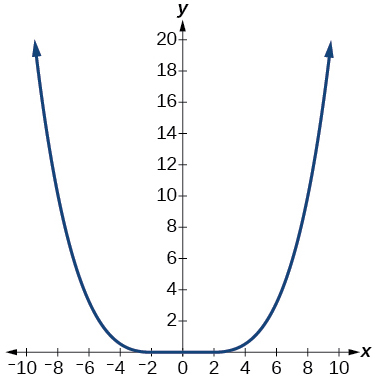even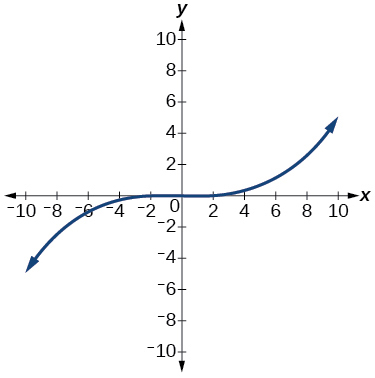## Absolute Value Functions

For the following exercises, write an equation for the transformation of $\text{\hspace{0.17em}}f\left(x\right)=|x|.$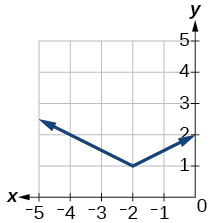$f\left(x\right)=\frac{1}{2}|x+2|+1$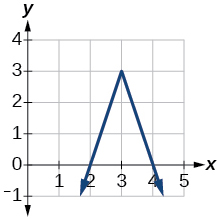$f\left(x\right)=-3|x-3|+3$

For the following exercises, graph the absolute value function.

$f\left(x\right)=|x-5|$

$f\left(x\right)=-|x-3|$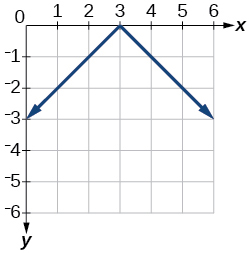$f\left(x\right)=|2x-4|$

## Inverse Functions

For the following exercises, find for each function.

$f\left(x\right)=9+10x$

$f\left(x\right)=\frac{x}{x+2}$

${f}^{-1}\left(x\right)=\frac{-2x}{x-1}$

For the following exercise, find a domain on which the function is one-to-one and non-decreasing. Write the domain in interval notation. Then find the inverse of restricted to that domain.

$f\left(x\right)={x}^{2}+1$

Given $f\left(x\right)={x}^{3}-5$ and $g\left(x\right)=\sqrt{x+5}:$

1. Find and $g\left(f\left(x\right)\right).$
2. What does the answer tell us about the relationship between $f\left(x\right)$ and $g\left(x\right)?$
1. and $g\left(f\left(x\right)\right)=x.$
2. This tells us that $f$ and $g$ are inverse functions

For the following exercises, use a graphing utility to determine whether each function is one-to-one.

$f\left(x\right)=\frac{1}{x}$

The function is one-to-one.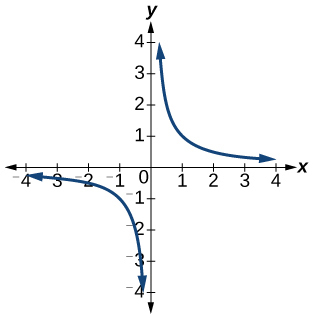$f\left(x\right)=-3{x}^{2}+x$

The function is not one-to-one.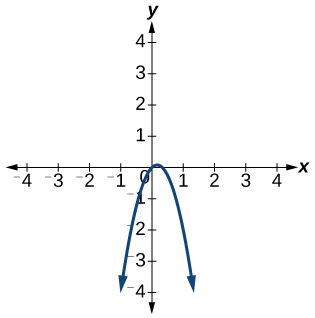If $f\left(5\right)=2,$ find ${f}^{-1}\left(2\right).$

$5$

If $f\left(1\right)=4,$ find ${f}^{-1}\left(4\right).$

## Practice test

For the following exercises, determine whether each of the following relations is a function.

$y=2x+8$

The relation is a function.

$\left\{\left(2,1\right),\left(3,2\right),\left(-1,1\right),\left(0,-2\right)\right\}$

For the following exercises, evaluate the function $\text{\hspace{0.17em}}f\left(x\right)=-3{x}^{2}+2x\text{\hspace{0.17em}}$ at the given input.

$f\left(-2\right)$

−16

$\text{\hspace{0.17em}}f\left(a\right)\text{\hspace{0.17em}}$

Show that the function $\text{\hspace{0.17em}}f\left(x\right)=-2{\left(x-1\right)}^{2}+3\text{\hspace{0.17em}}$ is not one-to-one.

The graph is a parabola and the graph fails the horizontal line test.

Write the domain of the function $\text{\hspace{0.17em}}f\left(x\right)=\sqrt{3-x}\text{\hspace{0.17em}}$ in interval notation.

Given $\text{\hspace{0.17em}}f\left(x\right)=2{x}^{2}-5x,\text{\hspace{0.17em}}$ find $f\left(a+1\right)-f\left(1\right)\text{\hspace{0.17em}}$ in simplest form.

$2{a}^{2}-a$

Graph the function

Find the average rate of change of the function $\text{\hspace{0.17em}}f\left(x\right)=3-2{x}^{2}+x\text{\hspace{0.17em}}$ by finding $\text{\hspace{0.17em}}\frac{f\left(b\right)-f\left(a\right)}{b-a}\text{\hspace{0.17em}}$ in simplest form.

$-2\left(a+b\right)+1$

For the following exercises, use the functions to find the composite functions.

$\left(g\circ f\right)\left(x\right)$

$\left(g\circ f\right)\left(1\right)$

$\sqrt{2}$

Express $\text{\hspace{0.17em}}H\left(x\right)=\sqrt{5{x}^{2}-3x}\text{\hspace{0.17em}}$ as a composition of two functions, $\text{\hspace{0.17em}}f\text{\hspace{0.17em}}$ and $\text{\hspace{0.17em}}g,\text{\hspace{0.17em}}$ where $\text{\hspace{0.17em}}\left(f\circ g\right)\left(x\right)=H\left(x\right).$

For the following exercises, graph the functions by translating, stretching, and/or compressing a toolkit function.

$f\left(x\right)=\sqrt{x+6}-1$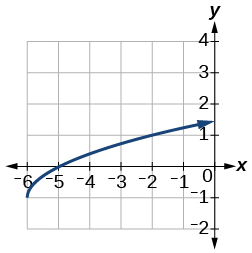$f\left(x\right)=\frac{1}{x+2}-1$

For the following exercises, determine whether the functions are even, odd, or neither.

$f\left(x\right)=-\frac{5}{{x}^{2}}+9{x}^{6}$

$\text{even}$

$f\left(x\right)=-\frac{5}{{x}^{3}}+9{x}^{5}$

$f\left(x\right)=\frac{1}{x}$

$\text{odd}$

Graph the absolute value function $\text{\hspace{0.17em}}f\left(x\right)=-2|x-1|+3.$

For the following exercises, find the inverse of the function.

$f\left(x\right)=3x-5$

${f}^{-1}\left(x\right)=\frac{x+5}{3}$

$f\left(x\right)=\frac{4}{x+7}$

For the following exercises, use the graph of $\text{\hspace{0.17em}}g\text{\hspace{0.17em}}$ shown in [link] .

On what intervals is the function increasing?

On what intervals is the function decreasing?

Approximate the local minimum of the function. Express the answer as an ordered pair.

$\left(1.1,-0.9\right)$

Approximate the local maximum of the function. Express the answer as an ordered pair.

For the following exercises, use the graph of the piecewise function shown in [link] .

Find $\text{\hspace{0.17em}}f\left(2\right).$

$f\left(2\right)=2$

Find $\text{\hspace{0.17em}}f\left(-2\right).$

Write an equation for the piecewise function.

$f\left(x\right)=\left\{\begin{array}{c}|x|\text{\hspace{0.17em}}\text{\hspace{0.17em}}\text{\hspace{0.17em}}\text{if}\text{\hspace{0.17em}}\text{\hspace{0.17em}}x\le 2\\ 3\text{\hspace{0.17em}}\text{\hspace{0.17em}}\text{\hspace{0.17em}}\text{\hspace{0.17em}}\text{\hspace{0.17em}}\text{if}\text{\hspace{0.17em}}\text{\hspace{0.17em}}x>2\end{array}$

For the following exercises, use the values listed in [link] .

$x$ $F\left(x\right)$
0 1
1 3
2 5
3 7
4 9
5 11
6 13
7 15
8 17

Find $\text{\hspace{0.17em}}F\left(6\right).$

Solve the equation $\text{\hspace{0.17em}}F\left(x\right)=5.$

$x=2$

Is the graph increasing or decreasing on its domain?

Is the function represented by the graph one-to-one?

yes

Find $\text{\hspace{0.17em}}{F}^{-1}\left(15\right).$

Given $\text{\hspace{0.17em}}f\left(x\right)=-2x+11,\text{\hspace{0.17em}}$ find $\text{\hspace{0.17em}}{f}^{-1}\left(x\right).$

${f}^{-1}\left(x\right)=-\frac{x-11}{2}$

bsc F. y algebra and trigonometry pepper 2
given that x= 3/5 find sin 3x
4
DB
remove any signs and collect terms of -2(8a-3b-c)
-16a+6b+2c
Will
Joeval
(x2-2x+8)-4(x2-3x+5)
sorry
Miranda
x²-2x+9-4x²+12x-20 -3x²+10x+11
Miranda
x²-2x+9-4x²+12x-20 -3x²+10x+11
Miranda
(X2-2X+8)-4(X2-3X+5)=0 ?
master
The anwser is imaginary number if you want to know The anwser of the expression you must arrange The expression and use quadratic formula To find the answer
master
The anwser is imaginary number if you want to know The anwser of the expression you must arrange The expression and use quadratic formula To find the answer
master
Y
master
master
Soo sorry (5±Root11* i)/3
master
Mukhtar
explain and give four example of hyperbolic function
What is the correct rational algebraic expression of the given "a fraction whose denominator is 10 more than the numerator y?
y/y+10
Mr
Find nth derivative of eax sin (bx + c).
Find area common to the parabola y2 = 4ax and x2 = 4ay.
Anurag
A rectangular garden is 25ft wide. if its area is 1125ft, what is the length of the garden
to find the length I divide the area by the wide wich means 1125ft/25ft=45
Miranda
thanks
Jhovie
What do you call a relation where each element in the domain is related to only one value in the range by some rules?
A banana.
Yaona
given 4cot thither +3=0and 0°<thither <180° use a sketch to determine the value of the following a)cos thither
what are you up to?
nothing up todat yet
Miranda
hi
jai
hello
jai
Miranda Drice
jai
aap konsi country se ho
jai
which language is that
Miranda
I am living in india
jai
good
Miranda
what is the formula for calculating algebraic
I think the formula for calculating algebraic is the statement of the equality of two expression stimulate by a set of addition, multiplication, soustraction, division, raising to a power and extraction of Root. U believe by having those in the equation you will be in measure to calculate it
Miranda
state and prove Cayley hamilton therom
hello
Propessor
hi
Miranda
the Cayley hamilton Theorem state if A is a square matrix and if f(x) is its characterics polynomial then f(x)=0 in another ways evey square matrix is a root of its chatacteristics polynomial.
Miranda
hi
jai
hi Miranda
jai
thanks
Propessor
welcome
jai
What is algebra
algebra is a branch of the mathematics to calculate expressions follow.
Miranda
Miranda Drice would you mind teaching me mathematics? I think you are really good at math. I'm not good at it. In fact I hate it. 😅😅😅
Jeffrey
lolll who told you I'm good at it
Miranda
something seems to wispher me to my ear that u are good at it. lol
Jeffrey
lolllll if you say so
Miranda
but seriously, Im really bad at math. And I hate it. But you see, I downloaded this app two months ago hoping to master it.
Jeffrey
which grade are you in though
Miranda
oh woww I understand
Miranda
Jeffrey
Jeffrey
Miranda
how come you finished in college and you don't like math though
Miranda
gotta practice, holmie
Steve
if you never use it you won't be able to appreciate it
Steve
I don't know why. But Im trying to like it.
Jeffrey
yes steve. you're right
Jeffrey
so you better
Miranda
what is the solution of the given equation?
which equation
Miranda
I dont know. lol
Jeffrey
Miranda
JeffreyByByBy RhodesBy OpenStaxBy OpenStaxBy JavaChamp TeamBy OpenStaxBy Janet ForresterBy Joli JuliannaBy Brooke DelaneyBy OpenStaxBy Jonathan Long